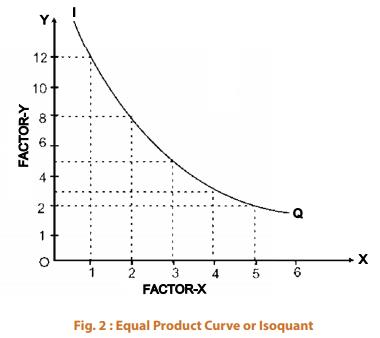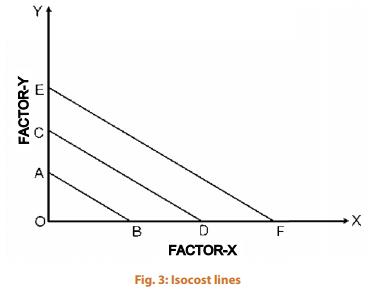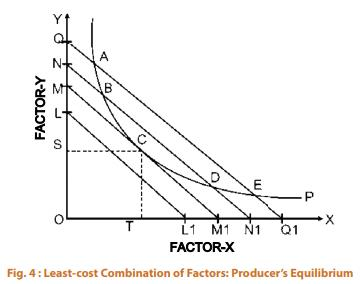Courses

# Production Optimisation CA Foundation Notes | EduRev

## Business Economics for CA Foundation

Created by: Sushil Kumar

## CA Foundation : Production Optimisation CA Foundation Notes | EduRev

The document Production Optimisation CA Foundation Notes | EduRev is a part of the CA Foundation Course Business Economics for CA Foundation.
All you need of CA Foundation at this link: CA Foundation

PRODUCTION OPTIMISATION
Normally, a profit maximising firm is interested to know what combination of factors of production (or inputs) would minimise its cost of production for a given output. This can be known by combining the firm’s production and cost functions, namely isoquants and isocost lines respectively.
Isoquants: Isoquants are similar to indifference curves in the theory of consumer behaviour. An isoquant represents all those combinations of inputs which are capable of producing the same level of output. Since an isoquant curve represents all those combination of inputs which yield an equal quantity of output, the producer is indifferent as to which combination he chooses. Therefore, Isoquants are also called equalproduct curves, production indifference curves or isoproduct curves. The concept of isoquant can be easily understood with the help of the following schedule
Table 2 : Various combinations of X and Y to produce a given level of output

 Factor combination Factor X Factor Y MRTS A 1 12 B 2 08 4 C 3 05 3 D 4 03 2 E 5 02 1

When we plot the various combinations of factor X and factor Y, we get a curve IQ as shown in Figure 2.Isoquants have properties similar to indifference curves. Isoquants are negatively sloped, convex to the origin due to diminishing marginal rate of technical substitution (MRTS) and are non intersecting. However, there is one important difference between the two: whereas in an indifference curve it is not possible to quantify the level of satisfaction acquired by the consumer, the level of production acquired by the producer is easily quantified. Thus, while isoquant IQ1 represents 100 units, curves IQ2, IQ3 etc. representing higher levels of production can be drawn. While a curve on the right represents a higher level of output, that on the left represents a lower level of output
Isocost or Equal-cost Lines: Isocost line, also known as budget line or the budget constraint line, shows the various alternative combinations of two factors which the firm can buy with given outlay. Suppose a firm has Rs. 1,000 to spend on the two factors X and Y. If the price of factor X is Rs. 10 and that of Y is Rs. 20, the firm can spend its outlay on X and Y in various ways. It can spend the entire amount on X and thus buy 100 units of X and zero units of Y or it can spend the entire outlay on Y and buy 50 units of it with zero units of X factor. In between, it can have any combination of X and Y. Whatever be the combination of factors the firm chooses, the total cost to the firm remains the same. In other words, all points on a budget line would cost the firm the same amount.
We can show the isocost line diagrammatically also. The X-axis shows the units of factor X and Y-axis the units of factor Y. When the entire ₹ 1,000 is spent on factor X, we get OB of factor X and when the entire amount is spent on factor Y we get OA of factor Y . The straight line AB which joins points A and B will pass through all combinations of factors X and Y which the firm can buy with outlay of  ₹ 1,000. The line AB is called isocost line.Figure 3 shows various isocost lines representing different combinations of factors with different outlays. Isoquants, which represent the technical conditions of production for a product and isocost lines which represent various ‘levels of cost or outlay’ (given the prices of two factors) can help the firm to optimize its production. It may try to minimise its cost for producing a given level of output or it may try to maximise the output for a given cost or outlay. Suppose the firm has already decided about the level of output to be produced. Then the question is with which factor combination the firm should try to produce the pre-decided level of output. The firm will try to use the least-cost combination of factors. The least cost combination of factors can be found by super-imposing the isoquant that represents the pre decided level of output on the isocost lines. This is shown in Figure 4.Suppose the rm has decided to produce 1,000 units (represented by iso-quant P). These units can be produced by any factor combination lying on P such as A, B, C, D, E, etc. The cost of producing 1,000 units would be minimum at the factor combination represented by point C where the iso-cost line MM1 is tangent to the given isoquant P. At all other points such as A, B, D, E the cost is more as these points lie on higher isocost lines Compared to MM1. Thus, the factor combination represented by point C is the optimum combination for the producer. It represents the least-cost of producing 1,000 units of output. It is thus clear that the tangency point of the given isoquant with an isocost line represents the least cost combination of factors for producing a given output.

 SUMMARY Production is the outcome of the combined activity of the four factors of production viz, land, labour, capital and organization. In simple terms production, means ‘creation of utility’. i.e. Utility of form, utility of place, utility of time and personal utility.Production does not include work done out of love and affection, voluntary services and goods produced for self consumption. Intention to exchange in the market is an essential component of production.Land includes all those free natural resources whose supply for the economy as a whole is fixed.Labour is all human efforts of body or of mind undergone partly or wholly with a view to secure an income apart from the pleasure derived directly from the work.Capital is that part of wealth of an individual or community which is used for further production of wealth. Capital, a stock concept, refers to produced means of production and it comprises of man-made machines and materials which are used for further production.Capital formation, also known as investment, means a sustained increase in the stock of real capital in a country. There are mainly three stages of capital formation viz. Savings which depends on ability to save and willingness to save; Mobilisation of savings which depends on availability of financial institutions and products; and Investment i.e. the process whereby the real savings get converted into real capital assets.Entrepreneur is the person who organises business; initiates production, remunerates other factors of production, introduces innovations and bears the risk and uncertainties of business.The objectives of an enterprise may be broadly categorised under the following heads. (i) Organic objectives (ii) Economic objectives (iii) Social objectives (iv) Human objectives (v) National objectives.An enterprise faces a number of problems from its inception, through its life time and till its closure. These may relate to objectives, location, size, physical facilities, finance, organization structure, marketing, legal formalities and industrial relations.Factors of production can be divided into two categories – Fixed factors are those factors whose quantity remains unchanged with changes in output within a capacity and variable factors are those the quantity of which change with a change in the level of output.Production function is the technical relationship between inputs and output. Samuelson describes production function as the relationship between the maximum amount of output that can be produced and the input required to make that output. It is defined for a given state of technology.The law of variable proportion or the law of diminishing returns is relevant when some factors are kept fixed and others are varied. It is applicable to the short-run.There are three stages of the law of variable proportion – where law of increasing returns, law of diminishing returns and law of negative returns operate.Total product is the total output resulting from the efforts of all the factors of production combined together at any time.Marginal product is the change in total product per unit change in the quantity of variable factor.Average product is the total product per unit of the variable factor.The Law of returns to scale describes the relationship between inputs and output in the long run when all inputs are changed in the same proportion. Returns to scale may be constant, increasing and decreasing.Constant returns to scale occur when the inputs increase by some proportion and the output also increases by the same proportion. It is also called linear homogeneous production function.Increasing returns to scale occur when the inputs increase by some proportion and the output increases more than proportionately.Decreasing returns to scale occur when the inputs increase by some proportion and the output increases less than proportionately.Isoquants or product indifference curves show all those combinations of different factors of production which give the same output to the producer.Isocost lines show various combinations of two factors which the firm can buy with given expenditure or outlay.By combining Isoquants and isocost lines, a producer can find out the combination of factors of production which is optimum i.e. the combination of factors of production which would minimise his cost of production.For producing a given output, the tangency point of the relevant isoquant (representing the output) with an isocost line represents the least cost combination of factors.

,

,

,

,

,

,

,

,

,

,

,

,

,

,

,

,

,

,

,

,

,

;